# 「Cryptography-MIT6875」: Lecture 14

In this series, I will learn MIT 6.875, Foundations of Cryptography, lectured by Vinod Vaikuntanathan.
Any corrections and advice are welcome. ^ - ^

Topics Covered:

• Perfect ZK Proof for QR Language
• Perfect ZK Proof for Graph Isomorphism
• Comp. ZK Proof for 3Coloring
• All NP Languages have Comp. ZK Proofs
• Commitment Schemes
Note:

The first two sections are also posted at the end of last Lecture, just for the continuous and complete description.

# ZK Definition

In the previous Lecture is introduced the definition of honest-verifier Zero-knowledge.

When the theorem is true, the view gives the honest verifier $V$ nothing that $V$ couldn’t have it on his own.

In real world, the verifier could be malicious that he can do anything he wants.

Refine the ZK definitions for any verifier $V^*$.

Recap:

A language $\mathcal{L}$ is actually a set of strings which represent true statements.
The view of $V^*$ is the transcripts and the coins, which contains all the messages going back and forth.

Perfect Zero-knowledge Definition:

An interactive Protocol $(P,V)$ is perfect zero-knowledge for a language $\mathcal{L}$ if for every PPT $V^*$, there exists a (expected) poly time simulator $S$ s.t. for every $x\in \mathcal{L}$, the following two distributions are identical:

• $\texttt{view}_{V^*}(P,V^*)$
• $\texttt{sim}_S(x,1^\lambda)$

Statistical Zero-knowledge Definition:

An interactive Protocol $(P,V)$ is statistical zero-knowledge for a language $\mathcal{L}$ if for every PPT $V^*$, there exists a (expected) poly time simulator $S$ s.t. for every $x\in \mathcal{L}$, the following two distributions are statistical indistinguishable:

• $\texttt{view}_{V^*}(P,V^*)$
• $\texttt{sim}_S(x,1^\lambda)$

Computational Zero-knowledge Definition:

An interactive Protocol $(P,V)$ is computational zero-knowledge for a language $\mathcal{L}$ if for every PPT $V^*$, there exists a (expected) poly time simulator $S$ s.t. for every $x\in \mathcal{L}$, the following two distributions are computationally indistinguishable:

• $\texttt{view}_{V^*}(P,V^*)$
• $\texttt{sim}_S(x,1^\lambda)$

# Perfect ZK Proof for QR Language

Recap the zero-knowledge proof for QR Language.

$\mathcal{L}=\{(N,y):y \textrm{ is a quadratic residue }\mod N\}$.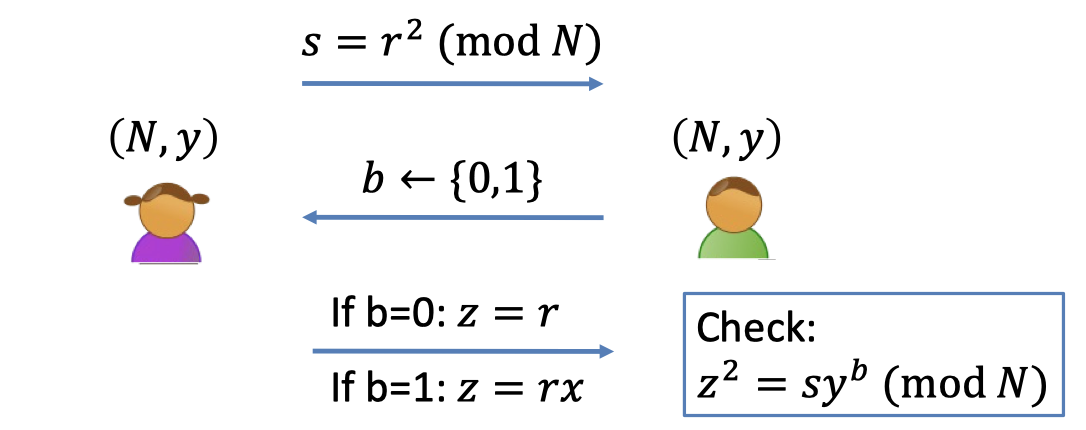We proved in last Lecture that the QR protocol is honest-verifier zero knowledge.

Now we only consider the malicious-verifier zero knowledge.

The QR protocol is malicious-verifier zero knowledge.

• The view of a malicious verifier $V^*$ is $\texttt{view}_{V^*}(P,V^*)=(s,b,z)$.
• When $V^*$ obtains the $s$, the only power that $V^*$ has is to choose the $b$ in a bizarre fashion rather than random.
• So the distribution of $b$ is not random.
• Let $b=V^*(s)$ denote the bizarre thing generated by $V^*$ after receiving $s$.
• The simulator $S$ only gets an instance of $(N,y)$ and wants to generate $\texttt{sim}_S((N,y),1^\lambda)=(s,b,y)$.

In order to produce the same distribution of $b$, the simulator $S$ needs to interact with the malicious verifier $V^*$.

It’s sort of a prover which also needs to interact with the verifier.

The only distinction is that the prover $P$ has to be online to answer the challenge from the verifier $V^*$ and the simulator $S$ can be offline with the goal of generating the view.

Claim:

The QR protocol is (malicious-verifier) perfect zero knowledge.

Simulator S works as follows:

1. First set $s=z^2/y^b$ for a random $z$ and a random $b$ and “feed” $s$ to $V^*$
2. Let $b'=V^*(s)$. (generated by $V^*$ in any bizarre fashion rather than random)
3. If $b=b’$, output $(s,b,z)$ and stop.
4. Otherwise, go back to step 1 and repeat. (also called “rewinding”)

Lemma:

1. $S$ runs in expected polynomial-time.
2. When $S$ outputs a view, it is identical to the view of $V^*$ in a real execution.

Lemma 1 is well proven.

The probability of terminating in one iteration is $1/2$ since $b$ is random.

So the expected iterations is $2$.

Proof of Lemma 2:

• We need to prove that the distribution of every component is identical.
• Real transcript: $\texttt{view}_{V^*}(P,V^*)=(s,b,z)$
• $s$ is square as same as random
• $b$ is generated in any bizarre way, i.e. $b=V^*(s)$.
• $z=\sqrt{sy^b}$ is random
• Simulated transcript: $\texttt{sim}_S((N,y),1^\lambda)=(s,b,y)$
• $s=z^2/y^b$ is square as same as random ($(N,y)\in \mathcal{L}$ so $y$ is square)
Besides, the distribution of $s$ hides $b$ perfectly.
• $b$ has the same distribution with $b’=V^*(s)$.
• $z$ is random
• QED

So far we have proven the QR protocol is (malicious verifier) zero-knowledge.

# ZK Proof for Graph Isomorphism

Recap the Graph Isomorphism Problem:Two graphs $G_0$ and $G_1$ are **isomorphic graphs** if they have the same number of vertices, edges and also the same edge connectivity.

The graph isomorphism problem is the computational problem of determining whether two finite graphs are isomorphic.

Now we describe the zero-knowledge proof for the claim that $G$ and $H$ are isomorphism graphs.The interaction protocol is as shown below: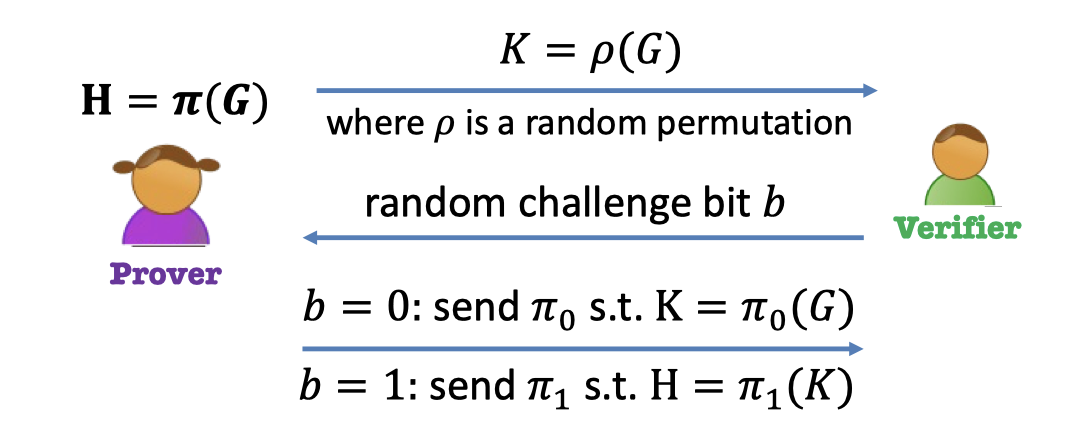Prover has the knowledge that she knows a map $\pi$ such that $H=\pi(G)$.

ZK Proof for Graph-iso:

1. Prover:
1. choose a random permutation $\rho$
2. generate a new random graph $K$ such that $K=\rho(G)$.
3. send the graph $K$ to verifier
2. Verifier: generate a random challenge bit $b$
3. Prover: has to answer the challenge bit
1. If $b=0$: Prover sends the map $\pi_0=\rho$ such that $K=\pi_0(G)$
(prove that she can map $G$ → $K$)
2. If $b=1$: Prover sends the map $\pi_1=\pi\circ \rho^{-1}$ such that $H=\pi_1(K)$
(prove that she can map $K$ → $H$)

Completeness:

Completeness is a property of the protocol when $P$ and $V$ are both honest.

We prove that the verifier will pass the equations and accept it.

• If $b=0$: check $K=\pi_0(G)=\rho(G)=K$.
• If $b=1$: check $H=\pi_1(K)=\pi\rho^{-1}(K)=\pi(G)=H$

Soundness:

Soundness is a property of the protocol when $P$ is malicious.

The verifier has to be sound because it has to work against an arbitrary $P$.

• Suppose $G$ and $H$ are non-isomorphic, and the prover could answer both the verifier challenges.
• Then the prover both prepares the $\pi_0$ and $\pi_1$ such that $K=\pi_0(G)$ and $H=\pi_1(K)$.
(Otherwise, she’ll be caught in half chance.)
• In other words, the prover can get $H=\pi_0\circ\pi_1(G)$, a contradiction.
• QED.

Perfect Zero Knowledge:

The following proof is my own deduction since the proof is omitted in the lecture.
Corrections and advice are welcome.
• The view of an arbitrary verifier $V^*$ is $\texttt{view}_{V^*}(P,V^*)=(K,b,\pi_b)$.
• When $V^*$ receives the graph $K$, the only power that $V^*$ has is to choose the $b$ in a bizarre fashion rather than random.
• So the distribution of $b$ is not random.
• Let $b=V^*(K)$ denote the bizarre thing generated by $V^*$ after receiving $K$.
• The simulator $S$ only gets an instance of $(G,H)$ and wants to generate $\texttt{sim}_S((G,H),1^\lambda)=(K,b,\pi_b)$.

Simulator S works as follows:

1. Pick a random permutation $\rho$ and a random $b$.
2. Generate a graph $K$ such that
1. If $b=0$: $K=\rho(G)$.
Let $\pi_0=\rho$.
2. If $b=1$: $K=\rho(H)$, i.e. $H=\rho^{-1}(K)$.
Let $\pi_1=\rho^{-1}$.
3. Feed the graph $K$ to verifier $V^*$
4. Let $b’=V^*(K)$
5. If $b=b’$, output $(K, b, \pi_b)$ and stop.
6. Otherwise, go back to step 1 and repeat.
Intuition of my proof:

It sort of split the $K$ into two intermediate points, $K_0$ and $K_1$.
The prover has the knowledge that could map $G$ to $K$ and map $K$ to $H$.
The point is that the simulator can simulate the knowledge that could map $G$ to $K_0$ and map $K_1$ to $H$.

Lemma:

1. $S$ runs in expected polynomial-time.
2. When $S$ outputs a view, it is identical to the view of $V^*$ in a real execution.

Lemma 1 is well proven.

The probability of terminating in one iteration is $1/2$ since $b$ is random.

So the expected iterations is $2$.

Proof of Lemma 2:

• We need to prove that the distribution of every component is identical.
• Real transcript: $\texttt{view}_{V^*}(P,V^*)=(K,b,\pi_b)$
• $K$ is a random graph since the random $\rho$.
• $b$ is generated in any bizarre way, i.e. $b=V^*(s)$.
• $\pi_b$ is a random map
• If $b=0$: $\pi_0=\rho$. → random map
• If $b=1$: $\pi_1=\pi\circ \rho^{-1}$. → random map.
• Simulated transcript: $\texttt{sim}_S((G,H),1^\lambda)=(K,b,\pi_b)$
• $\pi_b$ is a random map since $\rho$ is random.
• If $b=0$: $\pi_0=\rho$.
• If $b=1$: $\pi_1=\rho^{-1}$.
• $K$ is a random graph.
• If $b=0$, $K=\pi_0(G)=\rho(G)$
• If $b=1$, $K=\pi_1(H)=\rho^{-1}(H)$
• $b$ has the same distribution with $b’=V^*(s)$.
• QED

# Efficient Prover (given a Witness)

So far we keep saying that the prover is unbounded and the verifier is ppt.

But there are no unbounded people.

In both these protocols above, the (honest) prover is actually polynomial-time given the NP witness （the square root of $y$ in the case of QR, and the isomorphism in the case of graph-iso).

Therefore, the prover and the verifier can both be polynomial-time.

The only difference between the (honest) prover and the verifier is that the prover knows some privileged knowledge, a witness or a solution to a problem, that the verifier dose not know.

That’s the common way to reduce the zero-knowledge proofs.

The thing to point is that soundness is nevertheless against any, even computationally unbounded, prover $P^*$.

# All NP languages have Comp. ZK Proofs

We shows two languages with perfect ZK proofs, the QR protocol and the Graph-iso protocol.

• Do all NP languages have perfect ZK proofs ?
• The theorem[Fortnow’89, Aiello-Hastad’s 87] answered NO, unless bizarre stuff happens in complexity theory.
• Technically, the polynomial hierarchy collapses. That is NP = P.

Nevertheless, we can relax the question.

• Do all NP languages have ZK proofs ?

• Theorem: [Goldreich-Micali-Wigderson’87]

Assuming one-way permutations exist, all of NP has computational zero-knowledge proofs.

• It means that every language of NP problem has computational zero-knowledge proof given a witness.

Moreover, the assumption can be relaxed to one-way functions.

This theorem is amazing and it tells us that everything can be proved (in the sense of Euclid) can be proven in zero knowledge!

How to prove the theorem ? We cannot prove every NP problem one by one.

Luckily, we can prove NP-Complete Problem to which every other problem in NP can be reduced.

It turns out that there are a whole of complete problems.

There is a list of 20 odd problems that came up with in the 70s already and this list keeps increasing. So NP-complete problems is sort of a wealth.

We are going to pick the Graph Coloring Problem and prove it has Comp. ZK Proofs.

# ZK Proof for 3Coloring

Here is the Graph 3Coloring Problem.

Given a graph and three colors, red blue and green, you’re supposed to assign colors to every vertex such that no two adjacent vertexes have the same color.

(or every two adjacent vertexes have different colors)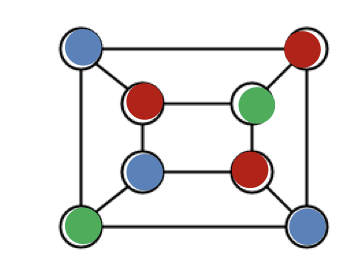Before proceeding to the zero-knowledge proof of the three-coloring, let’s introduce the lead-box model.

## The Lead-box Model

The lead-box model is as shown below.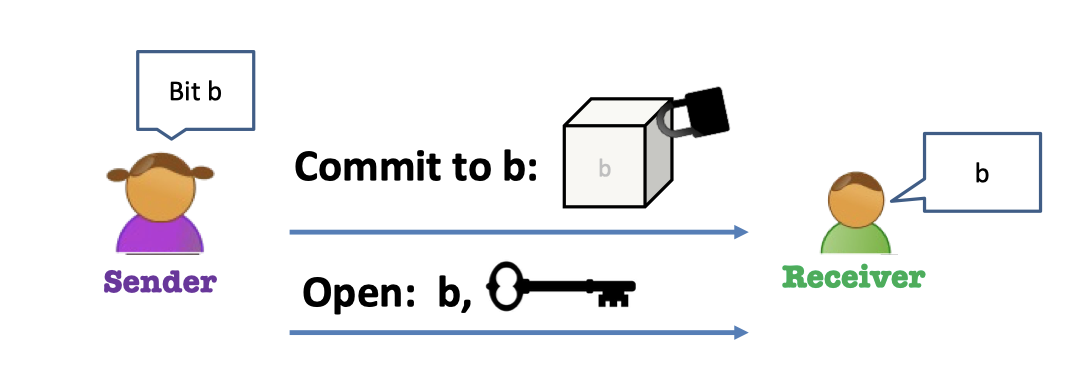• The sender Alice has a bit $b$.
1. Commit to $b$: put $b$ in a lead-box and locks it, and send the box to receiver.
2. Open $b$: send $b$ together with the key.
• Then the receiver Bob can check the thing in box is what Alice claims.

The lead-box above should be hiding and binding $b$.

Properties:

• Hiding means that the lead-box should completely hide $b$.
• Blinding means that the sender shouldn’t be able to open to $1-b$.

Once the Alice sends the box to Bob, she should not be able to change her mind about what’s inside the box.

That’ blinding. That’s a commitment.

It can be used for computational zero-knowledge.

It can also be used to ensure fairness.

We will later show how to implement such a lead-box (as a commitment protocol) using one-way permutations.

## ZK Proof with Lead-box: Part I

The language $\mathcal{L}$ is that the graph $G$ is 3-colorable.

Given a 3-colorable witness (solution), it can be proven in computational zero-knowledge.

The prover is given the graph $G$ and the 3-colorable witness.

The verifier is given the graph $G$.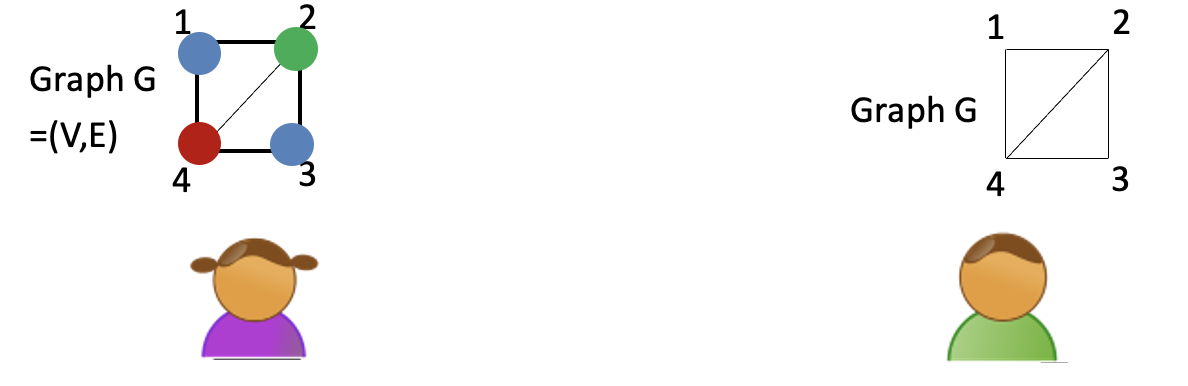The interaction of ZK proof is as shown below.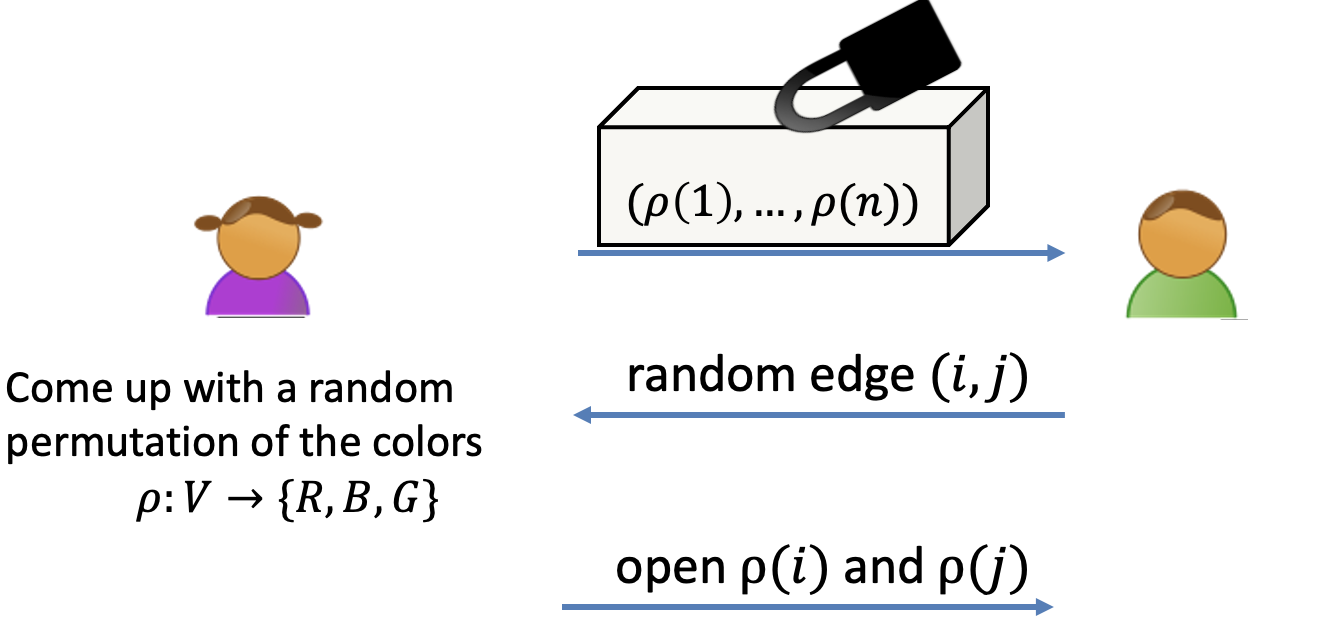Interactive Protocol for 3COL:

1. Prover: come up with a random permutation of the colors, $\rho:V\rightarrow \{R,G,B\}$.
The color of every vertex is masked by the random permutation.
2. Prover: commit to (the color of) every vertex.
3. Verifier: pick a random edge $(i,j)$
4. Prover: open $\rho(i)$ and $\rho(j)$
5. Verifier: check
1. Check the openings that $\rho(i)$ and $\rho(j)$ are what Alice claims.
2. Chek the $\rho(i),\rho(j)\in \{R,G,B\}$
3. Check: $\rho(i)\ne \rho(j)$

The completeness is well proven.

Soundness:

Soundness is the property of the protocol against dishonest prover $P$.

• If the graph is not 3COL, in every 3-coloring (that $P$ commits to), there is some edge whose end-points have the same color.
• $V$ will catch this edge and reject with probability $\ge 1/|E|$.
• In one time:
• the verifier accepts with probability $\le1-1/|E|$.
• Repeat $|E|\cdot \lambda$ times:
• he verifier accepts with probability $\le (1-1/|E|)^{|E|\cdot \lambda}\le 2^{-\lambda}$.
• which is negligible.
• QED

Moreover, the proof is Computational Zero-knowledge.

The key reason of zero-knowledge is the prover commits to all colors (of the vertices) but only open two colors (of the vertexes).

It leaks nothing to the verifier since the colors have been randomly permuted.

So the prover gives zero-knowledge to the verifier.

We will elaborate the Comp. Zero-knowledge in the following Part II.

More analysis into the first message(the message in the lead-box).

If the first message dose not exist, the proof is not sound.

The malicious prover can always answer “red” and “blue” because the verifier cannot check what Alice claims without the commitment.

If the first message are not in the lead-box, the proof is not zero-knowledge.

## Commitment Schemes

The lead-box is indeed a commitment protocol.

The Commitment Protocol $(S,R)$ works as follows.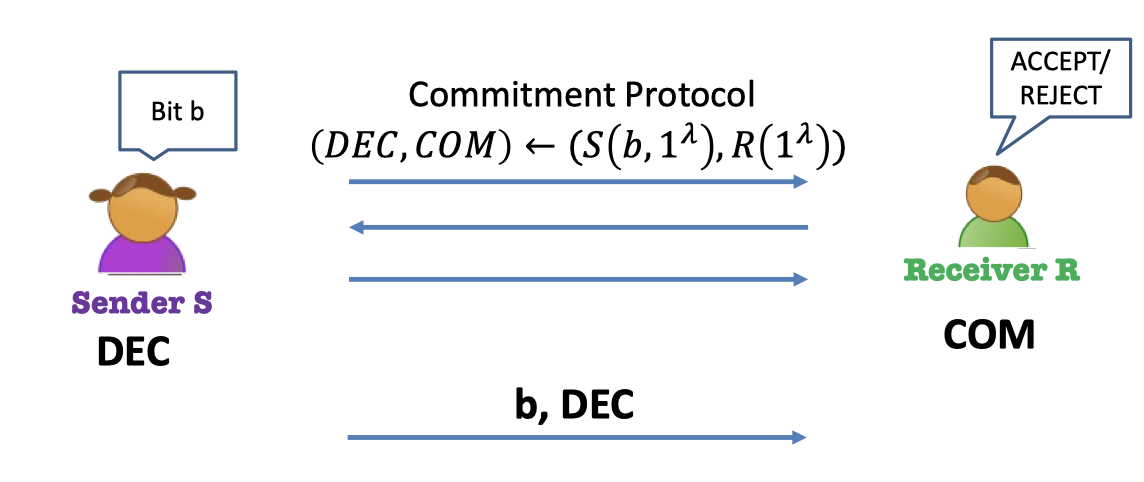Commitment Protocol $(S,R)$:

• There are two parties, sender $S$ and receiver $R$.
• Sender $S$ commits to a bit $b$, so the protocol is instanced to $(S(b,1^\lambda),R(1^\lambda))$.
• Let $\texttt{dec}$ be the sender’s output, decommitment.
• Let $\texttt{com}$ be the receiver’s output, commitment.
• Sender $S$ opens $b$
• $S$ sends $b$ together with $\texttt{dec}$.
• Receiver $R$ checks $b$ using $\texttt{dec}$.

Properties of Commitment Protocol:

1. Completeness: $R$ always accepts in an honest execution.
2. Computational Hiding:
• For every possibly malicious (PPT) $R^*$, $\texttt{view}_{R^*}(S(0),R^*)\approx_c\texttt{view}_{R^*}(S(1),R^*)$ (the view of $R^*$ is $(\texttt{com},b,\texttt{dec})$)
3. Perfect Binding:
• For every possibly malicious $S^*$, let $\texttt{com}$ be the receiver’s output in an execution of $(S^*, R)$.
• There is no pair of decommitments $(\texttt{dec}_0,\texttt{dec}_1)$ s.t. $R$ accepts both $(\texttt{com},0,\texttt{dec}_0)$ and $(\texttt{com},1,\texttt{dec}_1)$.

Completeness is the property of the commitment protocol when $S$ and $R$ are honest.

Computational Hiding is the property of commitment protocol against malicious $R^*$.

Perfect Binding is the property of commitment protocol against malicious $S^*$.

### A Commitment Scheme from any OWP

There is a commitment scheme starting from any OWP as follows.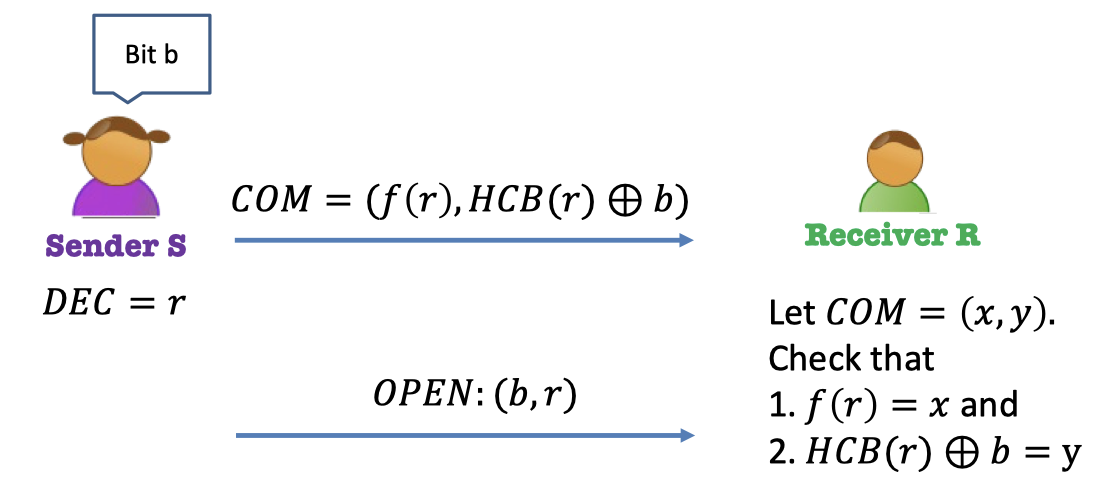Commitment Protocol $(S,R)$ from OWP:

• Sender $S$ commits to bit $b$

1. Pick a random $r$ as the decommitment, $\texttt{dec}=r$.
2. Compute the commitment $\texttt{com}=(f(r),HCB(r)\oplus b)$.
3. Send the commitment to $R$.
• Sender $S$ opens $b$

1. Send $(b,r)$ to $R$, $r$ as the $\texttt{dec}$.
• Receiver $R$ checks $b$ using $\texttt{dec}$.

• Let $\texttt{com}=(x,y)$
1. Check $f(r)=x$.
2. Check $HCB(r)\oplus b=y$.

This commitment scheme has completeness, comp. hiding and perfect binding.

Properties of Commitment Scheme:

• Completeness is well proven.
• Computational Hiding can be proven by the hardcore bit property.
• As for any arbitrary receiver $R^*$, it is hard to compute $HCB(r)$ given $f(r)$ since $f$ is OWP.
• We say that the hardcore bit of $f(r)$ computational hides the bit $b$.
• The point in Perfect Binding is that $f$ is a permutation.
• If $x=f(r)$ is fixed, then $r$ is fixed. There is no other $r’$ such that $f(r’)=f(r)$.
• Then $HCB(r)$ is fixed, and the bit $b$ is fixed since $y$ is fixed.
• That’s perfect binding.

## ZK Proof with Commitment: Part II

Replace the lead-box with the commitment protocol.

• Commitment to $\rho(k)$ is $\texttt{com}(\rho(k);r_k)$ where $r_k$ is the random.
• Decommitment to $\rho(k)$ is $r_k$.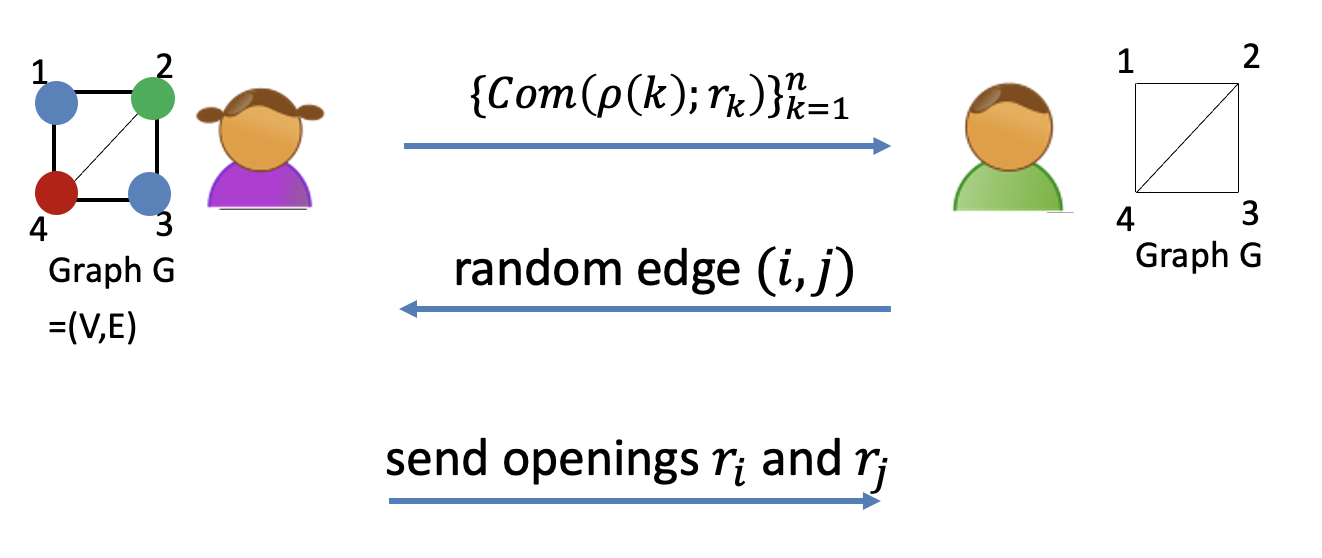Why is this protocol zero-knowledge?

Comp. Zero-knowledge:

We dive into a malicious-verifier zero-knowledge.

• What can an arbitrary verifier $V^*$ do ?
• He can see all these commitments to
• He can pick an arbitrary edge in bizarre fashion.
• Real transcript of malicious $V^*$: $\texttt{view}_{V^*}(P,V^*)=\left(\{\texttt{com}_k(\rho(k);r_k)\}_{k=1}^{n},(i,j),(\texttt{dec}_i,\texttt{dec}_j)\right)$.
• The commitment to every color (of the vertex): $\texttt{com}_k(\rho(k);r_k)$
• The edge chosen in bizarre fashion: $(i,j)=V^*(\{\texttt{com}_k\})$
• The decommitment: $(\texttt{dec}_i,\texttt{dec}_j)$.

Simulator S works as follows:

1. First pick a random edge $(i^*,j^*)$.
Color this edge with random, different colors.
Color all other edges red.
2. Feed the commitments of the colors to $V^*$ and get edge $(i,j)=V^*(\{\texttt{com}_k\})$.
3. If $(i,j)=(i^*,j^*)$, output the commitments and the openings $r_i$ and $r_j$ as the simulated transcript.
4. If $(i,j)\ne(i^*,j^*)$, go back to step 1 and repeat.

The key reason why it works is that the prover commits to all colors (of the vertices) but only open two colors (of the vertexes).
It leaks nothing to the verifier since the colors have been randomly permuted.

So the prover gives zero-knowledge to the verifier.

Lemma:

1. Assuming the commitments is hiding, $S$ runs in expected polynomial-time.
2. When $S$ outputs a view, it is computationally indistinguishable to the view of $V^*$ in a real execution.

Proof of Lemma 2:

Analysis the distribution of real transcript and simulated transcript.

• Real transcript: $\texttt{view}_{V^*}(P,V^*)=\left(\{\texttt{com}_k\}_{k=1}^{n},(i,j),(\texttt{dec}_i,\texttt{dec}_j)\right)$
• The commitments are computationally random since the computational hiding property.
• The distribution of $(i,j)$ is in bizarre fashion.
• The decommitments are random.
• Simulated transcript: $\texttt{sim}_{S}(G)=\left(\{\texttt{com}_k\}_{k=1}^{n},(i^*,j^*),(\texttt{dec}_i,\texttt{dec}_j)\right)$
• The commitments are computationally random since the computational hiding property.
• The distribution of $(i^*,j^*)$ is same as $(i,j)=V^*(\{\texttt{com}_k\})$.
• The decommitments are random.

## Examples of NP Assertions

• My public key is well-formed.
e.g. in RSA, prove the public key is $N$, a product of two primes together with an $e$ that is relatively to $\varphi(N)$.
• Encrypted bitcoin (or Zcash): “I have enough money to pay you.”
e.g. I will publish an encryption of my bank account and prove to you that my balance is $\ge \$X$. • Running programs on encrypted inputs: Given$Enc(x)$and$y$, prove that$y=\textrm{PROG}(x)\$.

「Cryptography-MIT6875」: Lecture 14

https://f7ed.com/2022/08/11/mit6875-lec14/

f1ed

2022-08-11

2022-08-15